Updating search results...

# 37 Results

View
Selected filters:
• NC.Math.6.G.1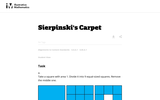Unrestricted Use
CC BY
Rating
0.0 stars
Overview:

This is a task from the Illustrative Mathematics website that is one part of a complete illustration of the standard to which it is aligned. Each task has at least one solution and some commentary that addresses important asects of the task and its potential use. Here are the first few lines of the commentary for this task: Take a square with area 1. Divide it into 9 equal-sized squares. Remove the middle one. What is the area of the figure now? Take the remaining 8 square...

Subject:
Mathematics
Material Type:
Activity/Lab
Provider:
Illustrative Mathematics
Provider Set:
Illustrative Mathematics
Author:
Illustrative Mathematics
08/06/2013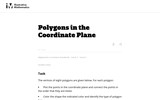Unrestricted Use
CC BY
Rating
5.0 stars
Overview:

This is a task from the Illustrative Mathematics website that is one part of a complete illustration of the standard to which it is aligned. Each task has at least one solution and some commentary that addresses important asects of the task and its potential use. Here are the first few lines of the commentary for this task: The vertices of eight polygons are given below. For each polygon: * Plot the points in the coordinate plane connect the points in the order that they a...

Subject:
Mathematics
Material Type:
Activity/Lab
Provider:
Illustrative Mathematics
Provider Set:
Illustrative Mathematics
Author:
Illustrative Mathematics
09/08/2013Unrestricted Use
CC BY
Rating
0.0 stars
Overview:

This is a task from the Illustrative Mathematics website that is one part of a complete illustration of the standard to which it is aligned. Each task has at least one solution and some commentary that addresses important aspects of the task and its potential use.

Subject:
Mathematics
Material Type:
Activity/Lab
Provider:
Illustrative Mathematics
Provider Set:
Illustrative Mathematics
Author:
Illustrative Mathematics
05/03/2019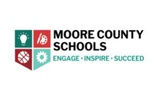Conditional Remix & Share Permitted
CC BY-NC
Rating
0.0 stars
Overview:

Students will create a map that includes (at minimum) five buildings, one must be your house and one must be the post office.   They will use the spheros to find the best route to the post office in order to ship their gift, in the shortest amount of time.  Students will need to find the length and width of each building on your map, using the spheros  and the distance=rate x time and find the area of each building.

Subject:
Mathematics
Material Type:
Activity/Lab
Author:
Carrie Robledo
10/15/2020Conditional Remix & Share Permitted
CC BY-NC-SA
Rating
0.0 stars
Overview:

This is an introductory project-based lesson. It is assumed that background knowledge of vocabulary, procedural steps, and concept have been previously instructed.  In this project, students will create a square-based pyramid net that would represent their actual tent. Then, they will need to figure out how much fabric that they will need in order to cover their tent.  Extensions are built into this activity that would allow the students to practice / explore skills with decimals, percents, and ratios.

Subject:
Mathematics
Material Type:
Activity/Lab
Author:
William Allred
Carrie Robledo
05/06/2021Conditional Remix & Share Permitted
CC BY-NC-SA
Rating
0.0 stars
Overview:

This parent guide supports parents in helping their child at home with the 6th grade Math content.

Subject:
Mathematics
Material Type:
Reference Material
Author:
AMBER GARVEY
01/05/2022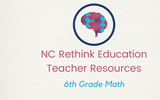Conditional Remix & Share Permitted
CC BY-NC-SA
Rating
0.0 stars
Overview:

This resource accompanies our Rethink 6th Grade Math course. It includes ideas for use, ways to support exceptional children, ways to extend learning, digital resources and tools, tips for supporting English Language Learners and students with visual and hearing impairments. There are also ideas for offline learning.

Subject:
Mathematics
Material Type:
Curriculum
Lesson Plan
Teaching/Learning Strategy
Author:
AMBER GARVEY
05/16/2022Conditional Remix & Share Permitted
CC BY-NC-SA
Rating
0.0 stars
Overview:

In this project, students will create videos that describe what surface area is, what algorithmic steps are needed to find surface area, and finally, they will show how to find the surface area of a polyhedron. Note: This lesson assumes that students have had previous lessons on surface area. This lesson is meant to be sort of a summative assessment tool in which students show their conceptual and procedural fluency of surface area.

Subject:
Mathematics
Material Type:
Activity/Lab
Author:
William Allred
Carrie Robledo
05/06/2021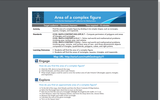Conditional Remix & Share Permitted
CC BY-NC-SA
Rating
0.0 stars
Overview:

Find the area of a complex figure by dividing it into simpler shapes, such as rectangles, squares, triangles, and trapezoids.

Subject:
Mathematics
Material Type:
Activity/Lab
Author:
ESRI
04/11/2020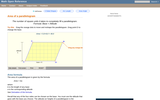Rating
0.0 stars
Overview:

A web page and interactive applet showing the ways to calculate the area of a parallelogram. The user can drag the vertices of the parallelogram and the other points change automatically to ensure it remains a parallelogram. A grid inside the shape allows students to estimate the area visually, then check against the actual computed area, which is continuously recomputed and displayed. The text on the page gives three different ways to calculate the area with a formula for each. The applet uses one of the methods to compute the area in real time, so it changes as the rhombus is reshaped with the mouse. A companion page is http://www.mathopenref.com/parallelogram.html showing the definition and properties of a parallelogram Applet can be enlarged to full screen size for use with a classroom projector. This resource is a component of the Math Open Reference Interactive Geometry textbook project at http://www.mathopenref.com.

Subject:
Geometry
Material Type:
Simulation
Provider:
Math Open Reference
Author:
John Page
05/07/2019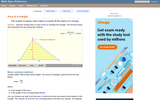Rating
5.0 stars
Overview:

An interactive applet and associated web page that explain the area of a triangle. The applet shows a triangle that can be reshaped by dragging any vertex. As it changes, the area is continually recalculated using the 'half base times height' method. The triangle has a fixed square grid in its interior that can be used to visually estimate the area for later correlation with the calculated value. The calculation can be hidden while estimation is in progress. The text page has links to a similar page that uses Heron's Formula to compute the area. Applet can be enlarged to full screen size for use with a classroom projector. This resource is a component of the Math Open Reference Interactive Geometry textbook project at http://www.mathopenref.com.

Subject:
Geometry
Material Type:
Simulation
Provider:
Math Open Reference
Author:
John Page
05/07/2019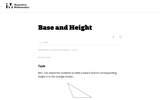Unrestricted Use
CC BY
Rating
0.0 stars
Overview:

The purpose of this task is to help students understand what is meant by a base and its corresponding height in a triangle and to be able to correctly identify all three base-height pairs.

Subject:
Mathematics
Material Type:
Activity/Lab
Provider:
Illustrative Mathematics
Provider Set:
Illustrative Mathematics
Author:
Illustrative Mathematics
05/01/2012Rating
0.0 stars
Overview:

This is an interactive lesson plan with videos, questions, and activities pertaining to area, surface area, and volume. It is a fun and engaging activity in which students pretend they are renovating their bedrooms and have to figure out how much of a material to by based on area measurments.

Subject:
Mathematics
Material Type:
Lesson Plan
Provider:
WOUB
02/26/2019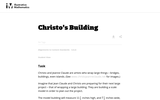Unrestricted Use
CC BY
Rating
0.0 stars
Overview:

This task is primarily about volume and surface area, although it also gives students an early look at converting between measurements in scale models and the real objects they correspond to.

Subject:
Mathematics
Material Type:
Activity/Lab
Provider:
Illustrative Mathematics
Provider Set:
Illustrative Mathematics
Author:
Illustrative Mathematics
05/01/2012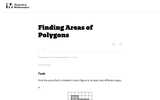Unrestricted Use
CC BY
Rating
5.0 stars
Overview:

The purpose of this task is to have students work on a sequence of area problems that shows the advantage of increasingly abstract strategies in preparation for developing general area formulas for parallelograms and triangles.

Subject:
Mathematics
Material Type:
Activity/Lab
Provider:
Illustrative Mathematics
Provider Set:
Illustrative Mathematics
Author:
Illustrative Mathematics
05/01/2012Rating
0.0 stars
Overview:

Students are given two vertices of a triangle and its area. They are asked to determine where the third vertex is located. The mathematics task is intended to be a problem or question that encourages the use of mathematical practices. The dialogue is meant to show how students might engage in the mathematical practices as they work on the task.

Subject:
Mathematics
Material Type:
Lesson Plan
Provider:
Education Development Center, Inc.
Author:
Education Development Center, Inc.
02/26/2019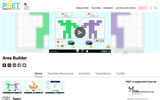Unrestricted Use
CC BY
Rating
0.0 stars
Overview:

Find the area of a shape by counting unit squares
Describe the relationship between area and perimeter
Build shapes with a given area and/or perimeter
Find the area of an irregular shape by decomposing into smaller, regular shapes (e.g., rectangles, triangles, squares)
Determine the scale factor of similar shapes
Generalize how area and perimeter change when scaling shapes

Subject:
Mathematics
Material Type:
Activity/Lab
Author:
PhET Interactive Simulations
10/01/2021Rating
0.0 stars
Overview:

Students will review coordinate plane concepts, practice map skills, review shapes, transform geometric figures, and calculate the area and perimeter of figures.

Subject:
Mathematics
Material Type:
Lesson Plan
Provider:
Alabama Learning Exchange
Author:
Aimee Smith
02/26/2019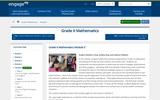Conditional Remix & Share Permitted
CC BY-NC-SA
Rating
0.0 stars
Overview:

In this module, students utilize their previous experiences in order to understand and develop formulas for area, volume, and surface area. Students use composition and decomposition to determine the area of triangles, quadrilaterals, and other polygons.

Subject:
Mathematics
Material Type:
Homework/Assignment
Author:
EnagageNY